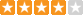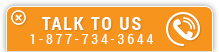# Digital Logical Circuits Volume I

Course Number: E-5007
Credit: 5 PDH
Subject Matter Expert: Lee Layton, P.E.
Price: \$149.75 Purchase using Reward Tokens. Details13 reviews
Overview

#### In Digital Logical Circuits Volume I, you'll learn ...

• Recognize different types of number systems as they relate to computers
• The concepts of most and least significant digits
• How to add and subtract in binary number systems
• The application of R’s complement in subtraction

#### OverviewPreview a portion of this course before purchasing it.

Credit: 5 PDH

Length: 64 pages

This 5-hour course is the first in a series of three courses about digital computer logic systems. This first course covers the basics of converting numbers into formats that are commonly used digital computer systems. Conversion to binary, octal, hexadecimal and BCD are covered.

The course begins with a fundamental review of the basic components of numbers, including determining the most significant digit and least significant digit in a number. From this basis, we move to adding and subtracting numbers in different bases, which is one of the fundamental computer logic operations. Conversions between bases are also covered as well as an explanation of binary coded decimal (BCD) numbers.

#### Specific Knowledge or Skill Obtained

This course teaches the following specific knowledge and skills:

• Recognize different types of number systems as they relate to computers
• The concepts of most and least significant digits
• How to add and subtract in binary number systems
• The application of R’s complement in subtraction
• Convert values from decimal into other number systems
• Convert values into Binary-coded decimal numbers
• How to add in binary-coded decimal

#### Certificate of Completion

You will be able to immediately print a certificate of completion after passing a multiple-choice quiz consisting of 30 questions. PDH credits are not awarded until the course is completed and quiz is passed.

Board Acceptance
 This course is applicable to professional engineers in: Alabama (P.E.) Alaska (P.E.) Arkansas (P.E.) Delaware (P.E.) Florida (P.E. Area of Practice) Georgia (P.E.) Idaho (P.E.) Illinois (P.E.) Illinois (S.E.) Indiana (P.E.) Iowa (P.E.) Kansas (P.E.) Kentucky (P.E.) Louisiana (P.E.) Maine (P.E.) Maryland (P.E.) Michigan (P.E.) Minnesota (P.E.) Mississippi (P.E.) Missouri (P.E.) Montana (P.E.) Nebraska (P.E.) Nevada (P.E.) New Hampshire (P.E.) New Jersey (P.E.) New Mexico (P.E.) New York (P.E.) North Carolina (P.E.) North Dakota (P.E.) Ohio (P.E. Self-Paced) Oklahoma (P.E.) Oregon (P.E.) Pennsylvania (P.E.) South Carolina (P.E.) South Dakota (P.E.) Tennessee (P.E.) Texas (P.E.) Utah (P.E.) Vermont (P.E.) Virginia (P.E.) West Virginia (P.E.) Wisconsin (P.E.) Wyoming (P.E.)
Reviews (13)
More DetailsPreview a portion of this course before purchasing it.

Credit: 5 PDH

Length: 64 pages